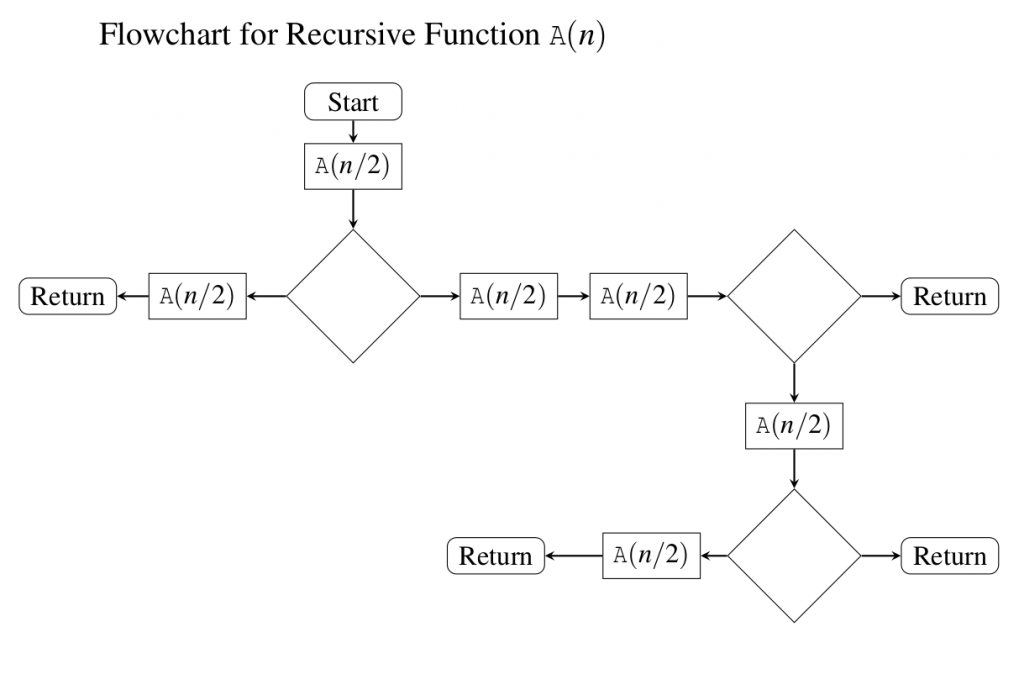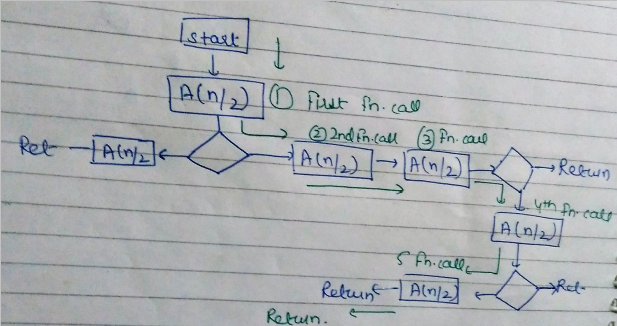# GATE | GATE-CS-2016 (Set 2) | Question 49

The given diagram shows the flowchart for a recursive function A(n). Assume that all statements, except for the recursive calls, have O(1) time complexity. If the worst case time complexity of this function is O(nα), then the least possible value (accurate up to two decimal positions) of α is __________(A) 2.2 to 2.4
(B) 3.2 to 3.4
(C) 0 to 1.8
(D) 1

Explanation: The time complexity of a recurrence relation is the worst case time complexity. First we have to find out the number of function calls in worst case from the flow chart.
The worst case will be when all the conditions (diamond boxes) turn out towards non-returning paths taking the longest root. In the longest path we have 5 function calls.So the recurrence relation will be –
A(n) = 5A(n/2) + O(1) (O(1) because rest of the statements have O(1) complexity.)
Solving this recurrence relation using Master theorem –
a = 5 , b= 2 , f(n) =O(1) , nlogb a= nlog2 5 (case 1 master theorem)
A(n) =nlogb a
value of log 25 is 2.3219, so, the best option is option a

This explanation has been contributed by Parul Sharma.

Quiz of this Question

My Personal Notes arrow_drop_up
Article Tags :

Be the First to upvote.

Please write to us at contribute@geeksforgeeks.org to report any issue with the above content.